# 时滞耦合惯性项神经系统的多混沌路径共存*

(1. 上海海洋大学 信息学院， 上海 201306；2. 上海海洋大学 食品学院， 上海 201306)

## 1 系统模型及其平衡点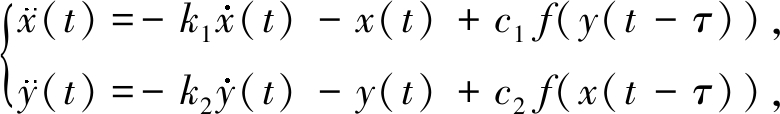(1)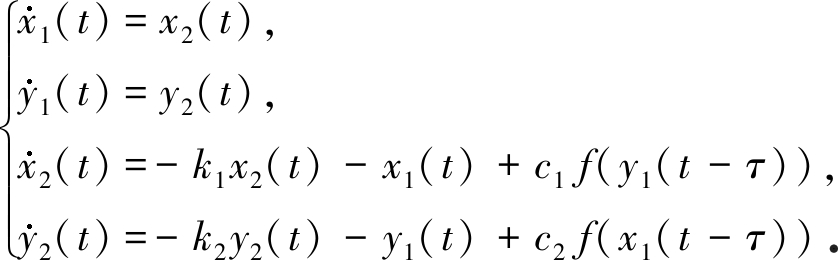(2)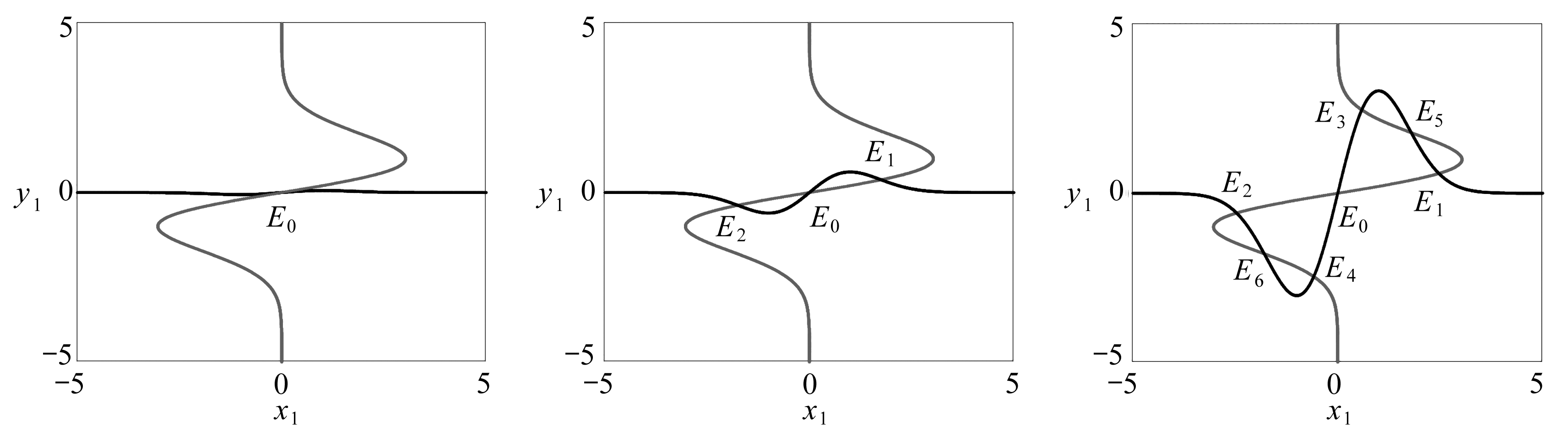(a) c2=0.1 (b) c2=1 (c) c2=5

Fig. 1 Intersection points of x1=c1 f(y1) and y1=c2 f(x1) showing the number of equilibrium for fixed c1=5 in neural system (2)

## 2 倍周期分岔序列共存

### 2.1 一级倍周期分岔序列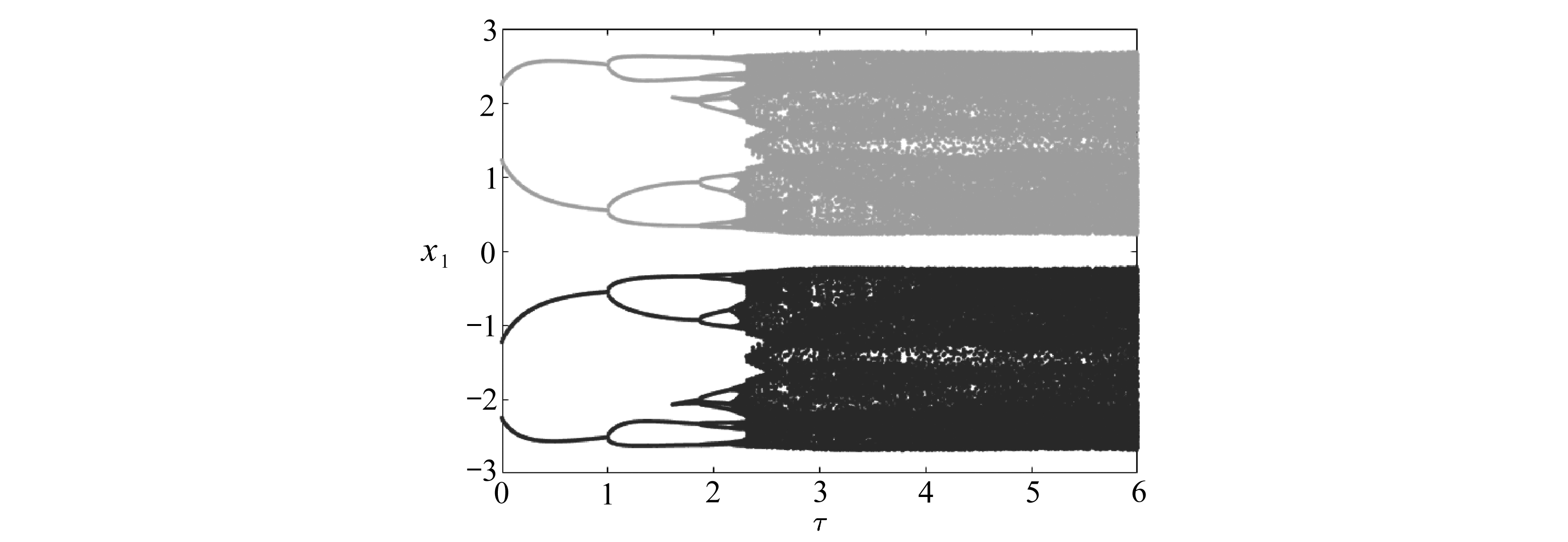Fig. 2 System (2) entering a pair of chaotic attractors for [0,6] through the 1st period-doubling bifurcation with initial conditions of (2,-2,0,0) and (-2,2,0,0)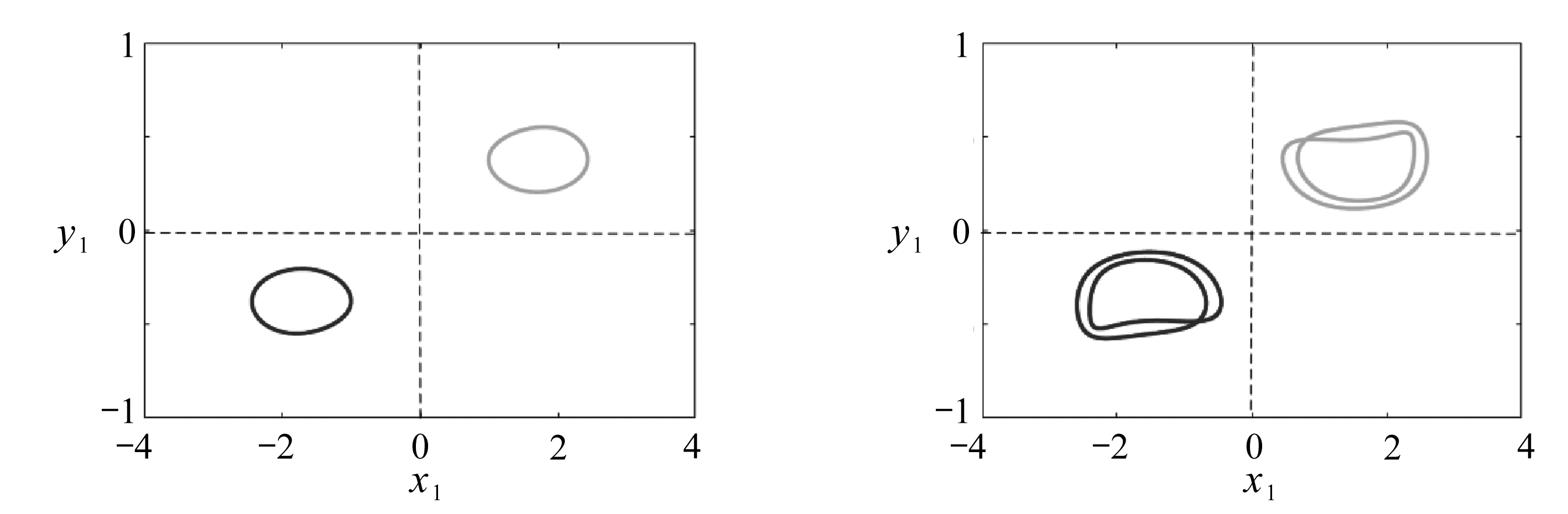(a) τ=0.1 (b) τ=1.05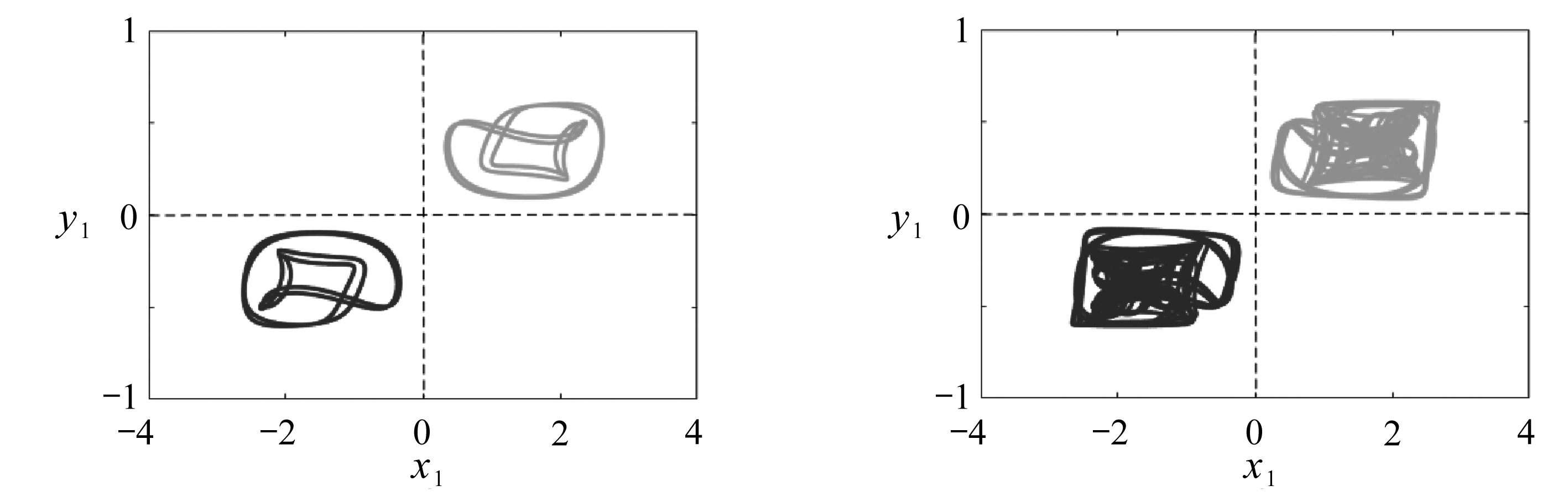(c) τ=2 (d) τ=3

Fig. 3 Phase portraits of the steady state for the 1st period-doubling bifurcationunder initial conditions of (2,-2,0,0) and (-2,2,0,0)

### 2.2 二级倍周期分岔序列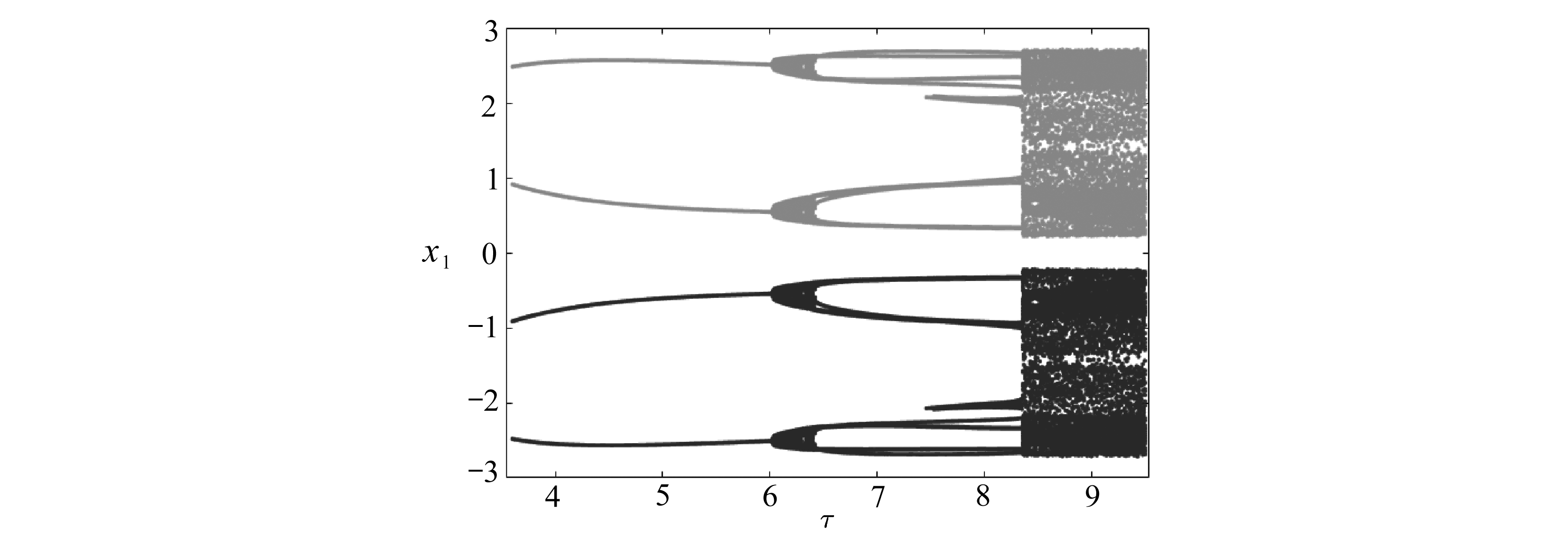Fig. 4 System (2) entering a pair of chaotic attractors for [3.54,9.54] through the 2nd period-doubling bifurcation with initial conditions of (0,2.5,0,0) and (0,-2.5,0,0)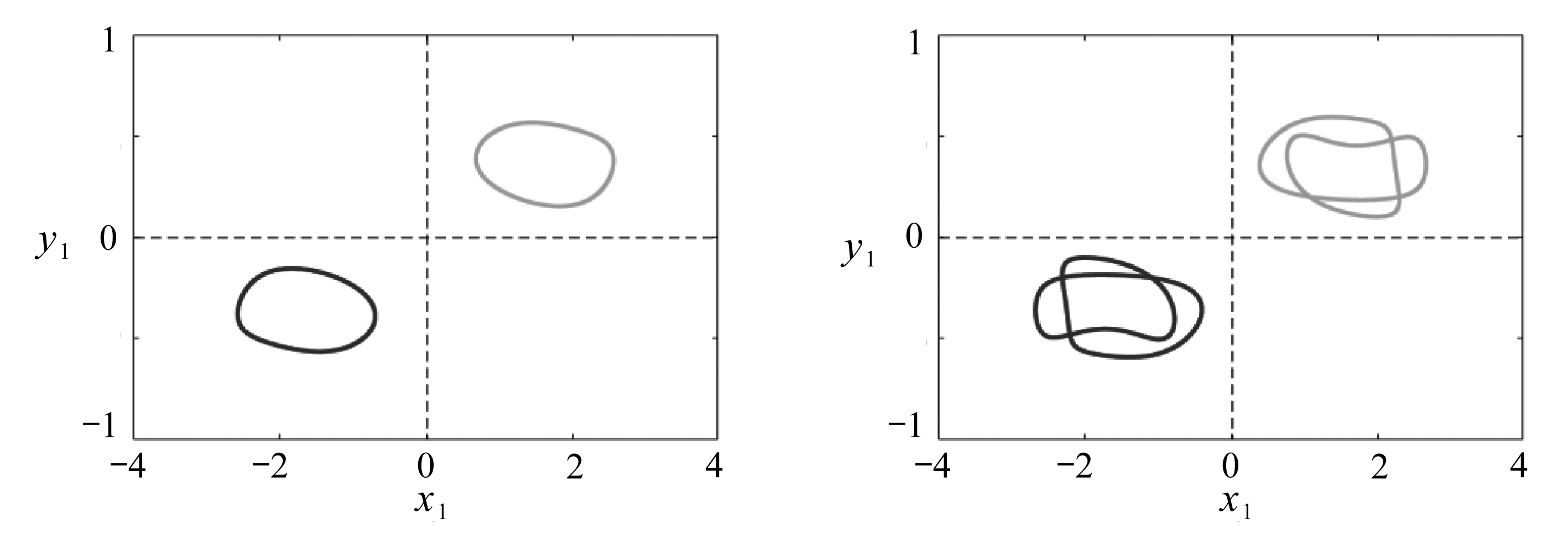(a) τ=4.4 (b) τ=6.7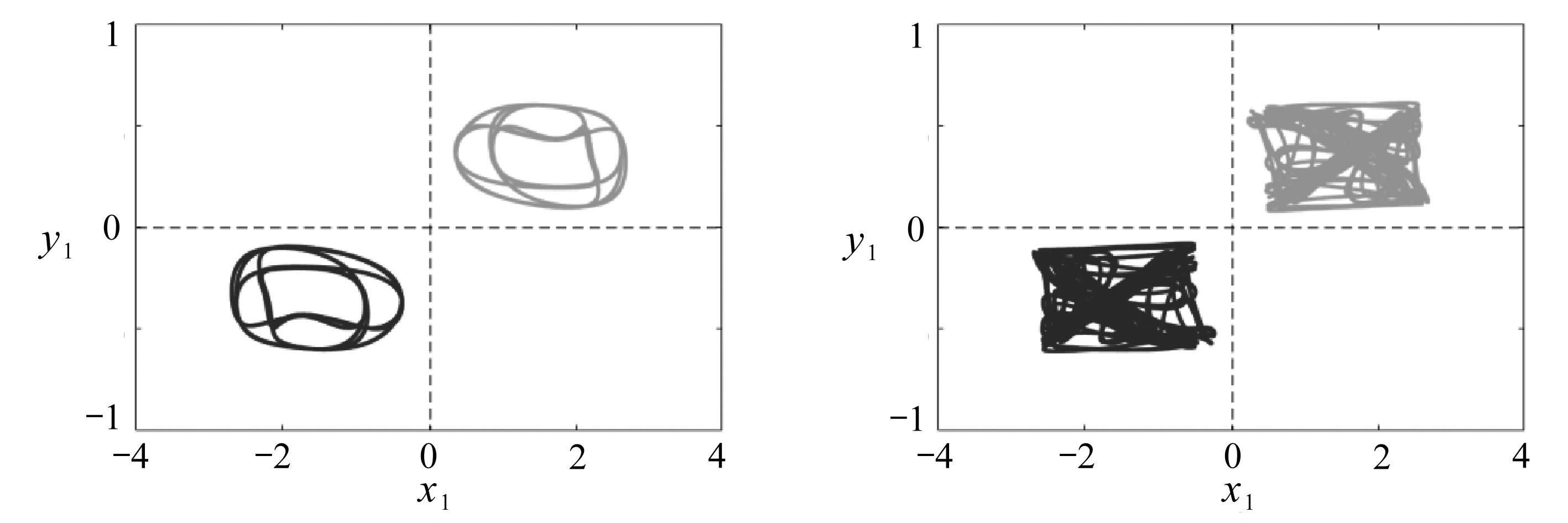(c) τ=7 (d) τ=9

Fig. 5 Phase portraits of the steady state for the 2nd period-doubling bifurcation under initial conditions of (0,2.5,0,0) and (0,-2.5,0,0)

## 3 概周期分岔序列共存

### 3.1 一级概周期分岔序列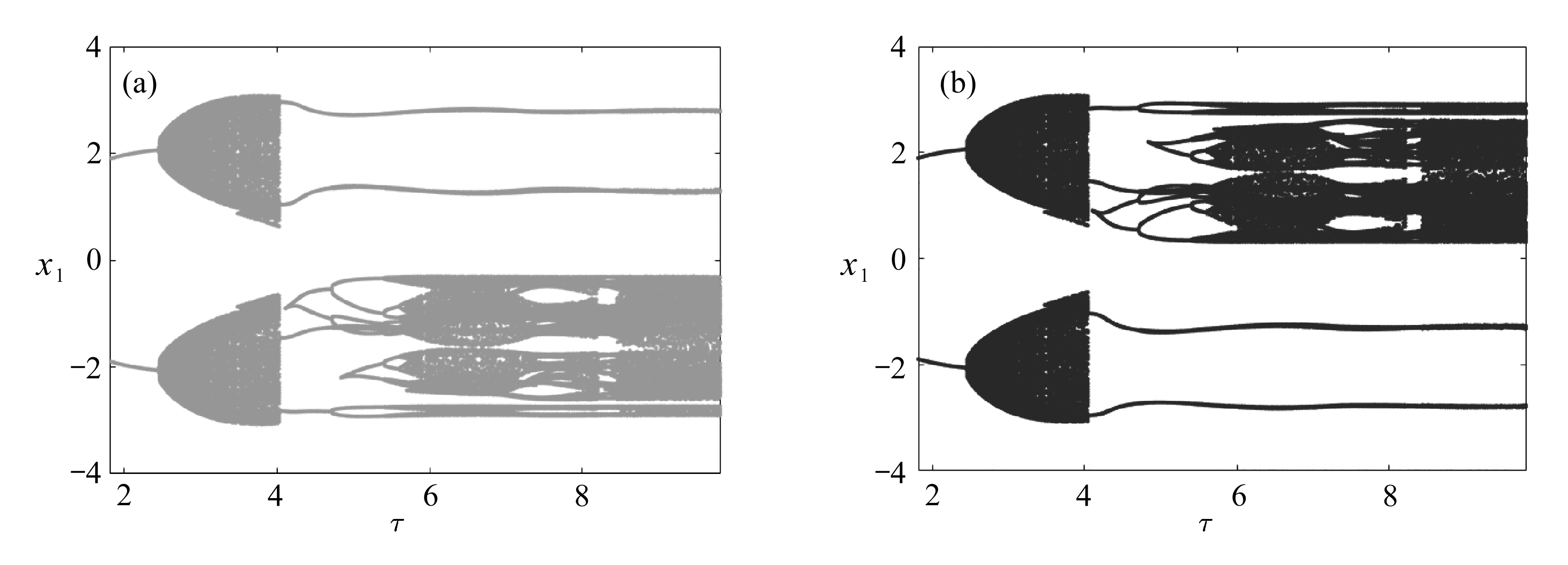Fig. 6 The 1st coexistence of quasi-periodic bifurcations for system (2) in [1.81,9.81] with initial conditions of (1.8,-2.4,0,0) and (-1.8,2.4,0,0)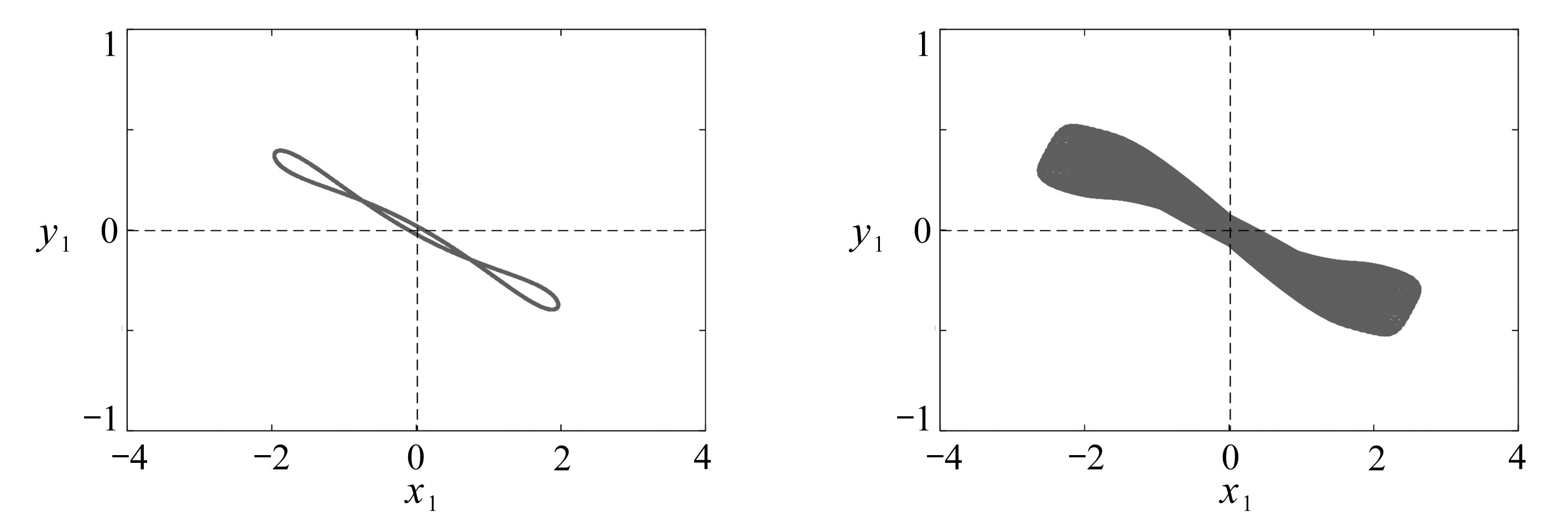(a) τ=2 (b) τ=2.7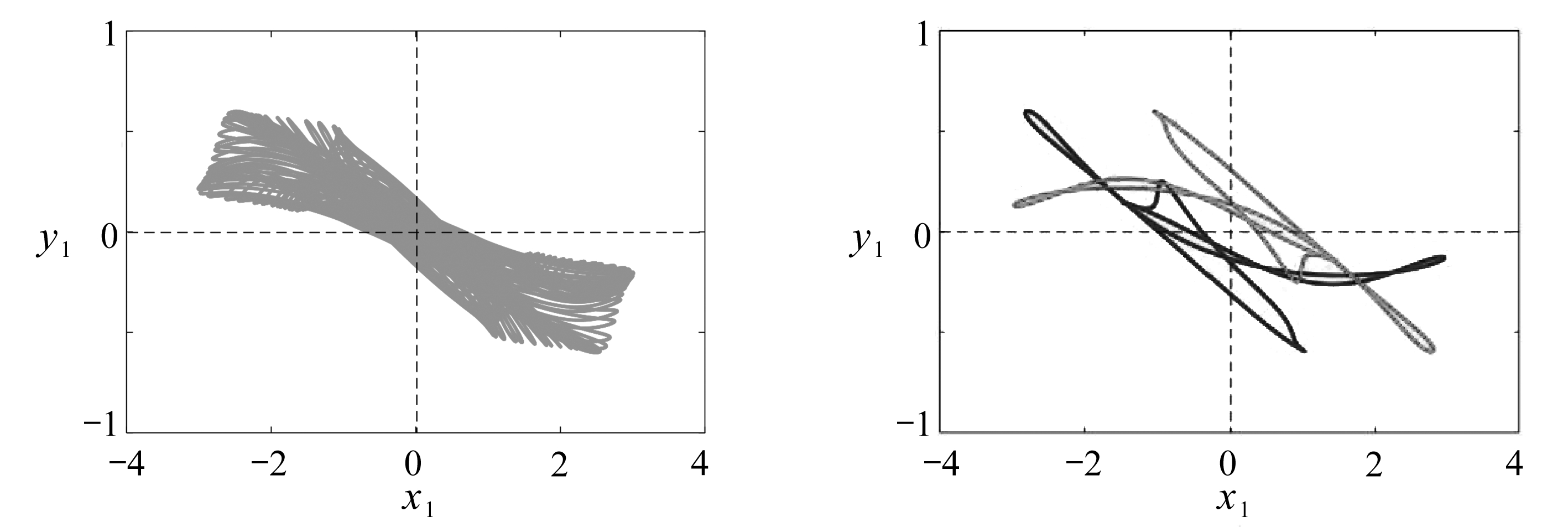(c) τ=3.3 (d) τ=4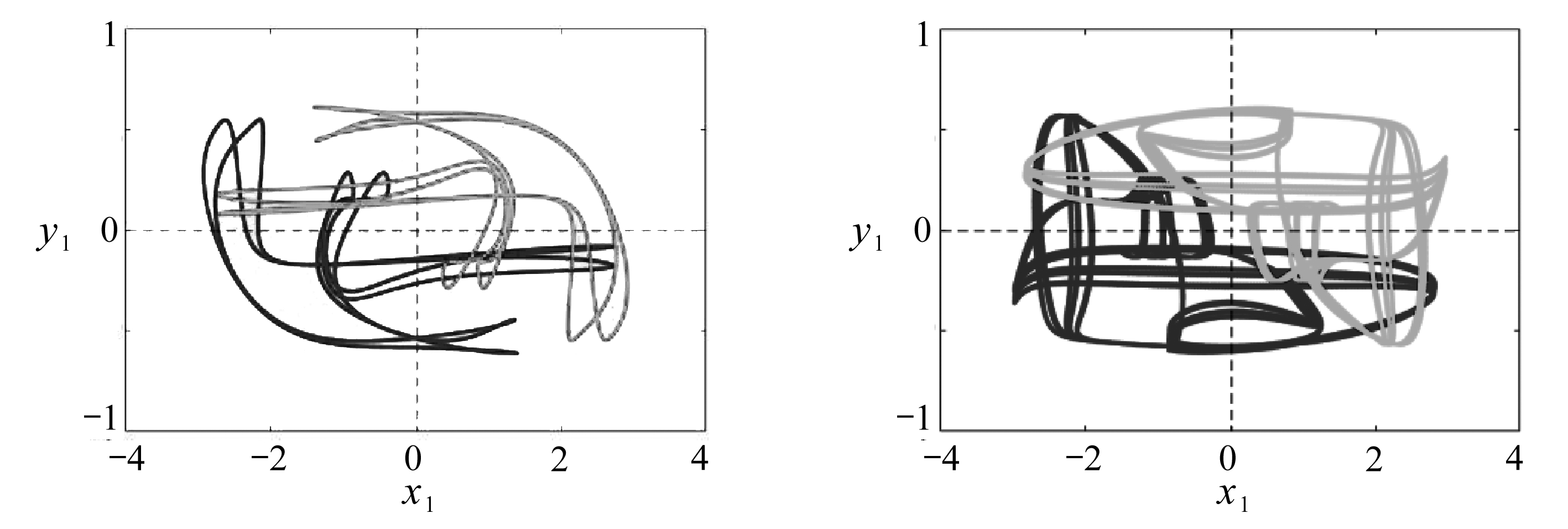(e) τ=5 (f) τ=7

Fig. 7 Phase portraits of the steady state for the 1st quasi-periodic bifurcation under initial conditions of (1.8,-2.4,0,0) and (-1.8,2.4,0,0)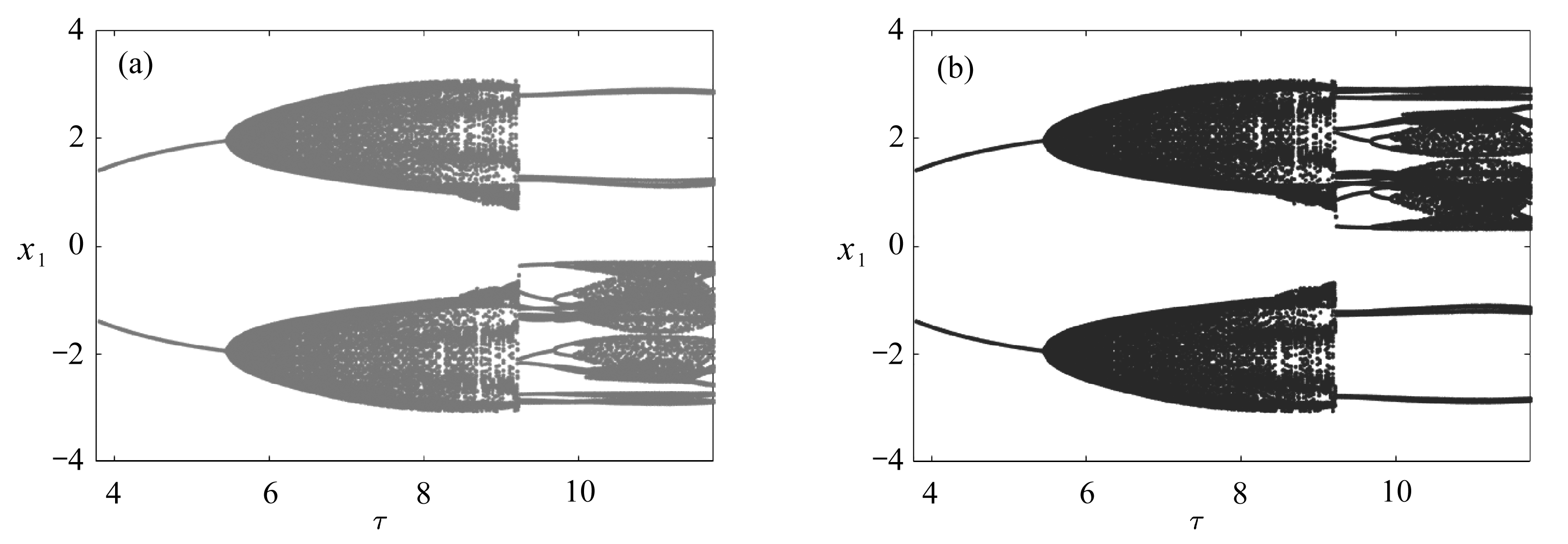Fig. 8 The 2nd coexistence of quasi-periodic bifurcations for system (2) in [3.75,11.75] with the initial conditions of (3,-3,0,0) and (-3,3,0,0)

### 3.2 二级概周期分岔序列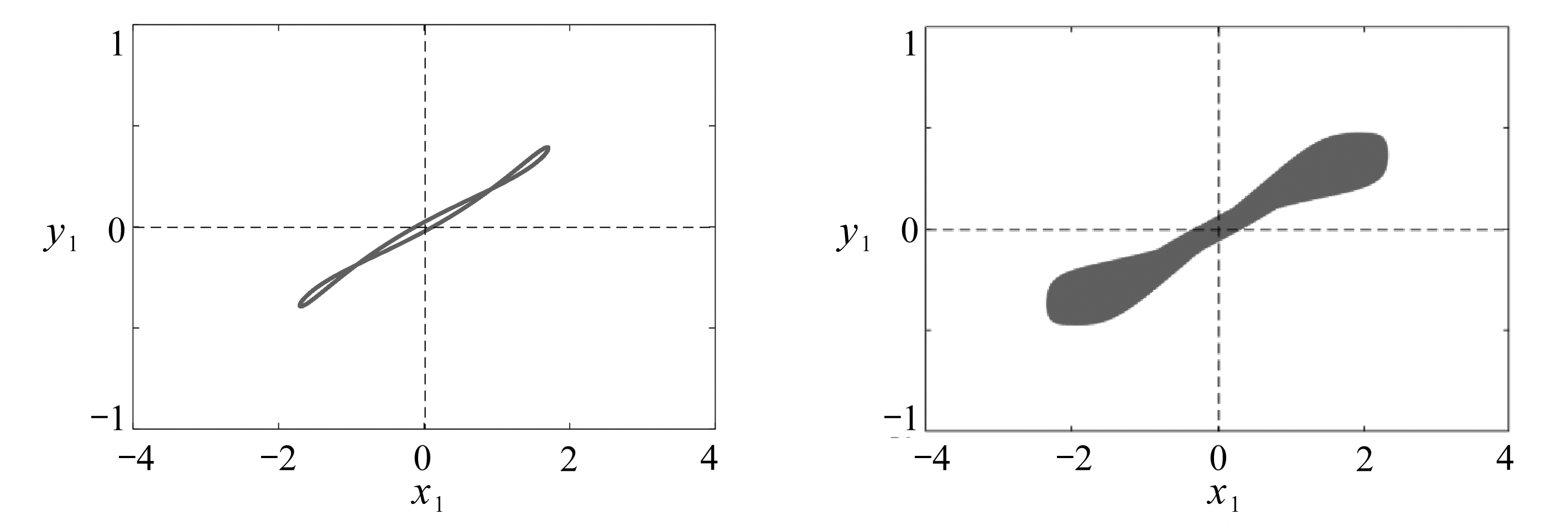(a) τ=4.5 (b) τ=5.7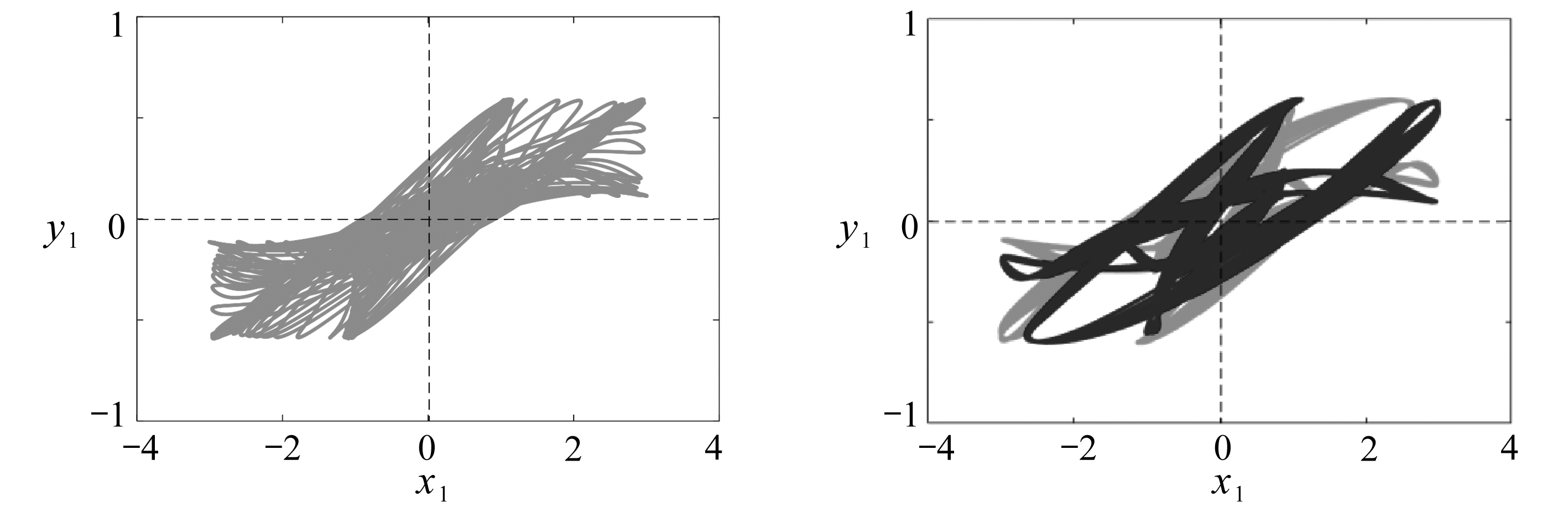(c) τ=8 (d) τ=9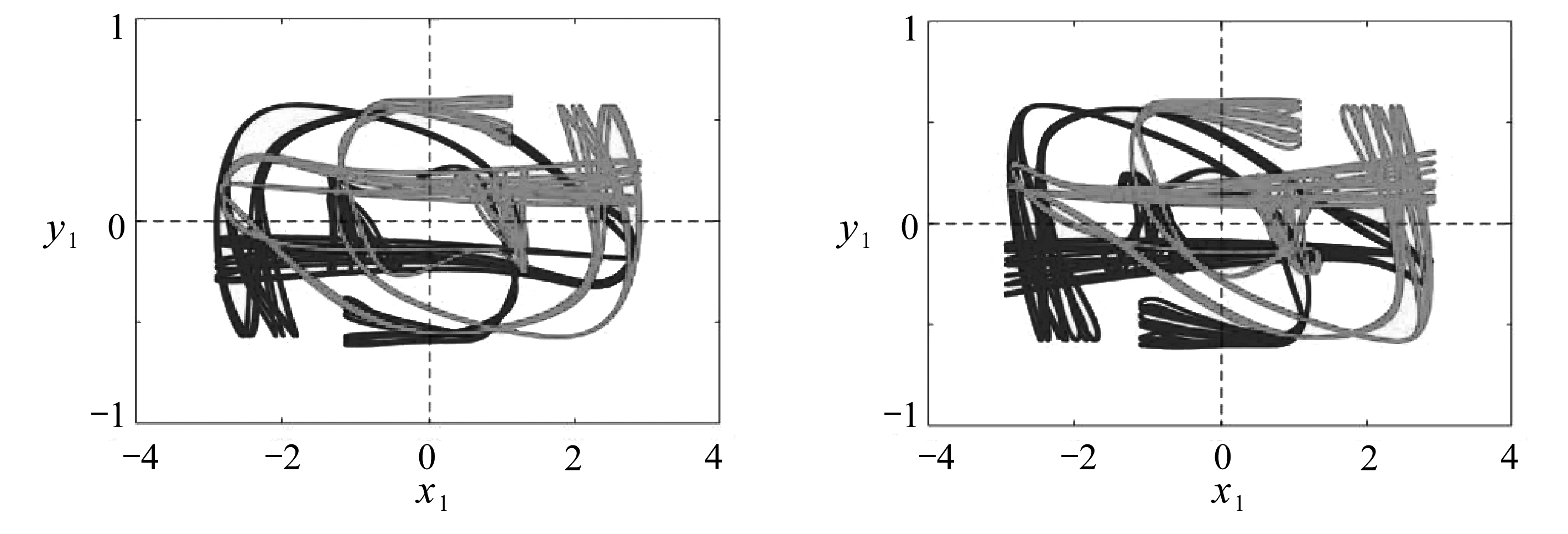(e) τ=10 (f) τ=11

Fig. 9 Phase portraits of the steady state for the 2nd quasi-periodic bifurcation under initial conditions of (3,-3,0,0) and (-3,3,0,0)

## 4 结 论

 PISARCHIK A N, FEUDEL U. Control of multistability[J]. Physics Reports, 2014, 540(4): 167-218.

 AIHARA K, TAKABE T, TOYODA M. Chaotic neural networks[J]. Physics Letter A, 1990, 144(67): 333-340.

 HUANG W Z, HUANG Y. Chaos, bifurcation and robustness of a class of Hopfield neural networks[J]. International Journal of Bifurcation and Chaos, 2011, 21(3): 885-895.

 CHEN P F, CHEN Z Q, WU W J. A novel chaotic system with one source and two saddle-foci in Hopfield neural networks[J]. Chinese Physics B, 2010, 4: 134-139.

 PISARCHIK A N, JAIMES-REATEGUI R, GARC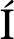A-VELLISCA M A. Asymmetry in electrical coupling between neurons alters multistable firing behavior[J]. Chaos, 2018, 28(3): 033605.

 CHENG C Y. Coexistence of multistability and chaos in a ring of discrete neural network with delays[J]. International Journal of Bifurcation and Chaos, 2010, 20(4): 1119-1136.

 SONG Z G, XU J, ZHEN B. Multitype activity coexistence in an inertial two-neuron system with multiple delays[J]. International Journal of Bifurcation and Chaos, 2015, 25(13): 1530040.

 LAI Q, HUANG J N. Coexistence of multiple attractors in a new chaotic system[J]. Acta Physica Polonica B: Particle Physics & Field, 2016, 47(10): 2315-2323.

 JIMENEZ-LOPEZ E, GONZALEZ SALAS J S, ONTANON-GARCIA L J, et al. Generalized multistable structure via chaotic synchronization and preservation of scrolls[J]. Journal of the Franklin Institute, 2013, 350(10): 2853-2866.

 MAURO A, CONTI F, DODGE F, et al. Subthreshold behavior and phenomenological impedance of the squid giant axon[J]. The Journal of General Physiology, 1970, 55: 497-523.

 ANGELAKI D E, CORREIA M J. Models of membrane resonance in pigeon semicircular canal type Ⅱ hair cells[J]. Biological Cybernetics, 1991, 65(1): 1-10.

 TANI J. Proposal of chaotic steepest descent method for neural networks and analysis of their dynamics[J]. Electronics and Communications in Japan (Part 3: Fundamental Electronic Science), 1992, 75(4): 62-70.

 BABCOCK K L, WESTERVELT R M. Dynamics of simple electronic neural networks[J]. Physica D, 1987, 28: 305-316.

 SONG Z G, XU J. Stability switches and Bogdanov-Takens bifurcation in an inertial two-neuron coupling system with multiple delays[J]. Science China Technological Sciences, 2014, 57: 893-904.

 SONG Z G, WANG C H, ZHEN B. Codimension-two bifurcation and multistability coexistence in an inertial two-neuron system with multiple delays[J]. Nonlinear Dynamics, 2016, 85: 2099-2113.

 YAO S W, DING L W, SONG Z G, et al. Two bifurcation routes to multiple chaotic coexistence in an inertial two-neural system with time delay[J]. Nonlinear Dynamics, 2019, 95: 1-15.

 CRESPI B. Storage capacity of non-monotonic neurons[J]. Neural Networks, 1999, 12(10): 1377-1389.

 LI C G, CHEN G R. Coexisting chaotic attractors in a single neuron model with adapting feedback synapse[J]. Chaos, Solitons & Fractals, 2005, 23: 1599-1604.

 LI Chunguang, CHEN Guangrong, LIAO Xiaofeng, et al. Hopf bifurcation and chaos in a single inertial neuron model with time delay[J]. The European Physical Journal B:Condensed Matter and Complex Systems, 2004, 41: 337-343.

# Multistage Coexistence of Different Chaotic Routes in a Delayed Neural System

LI Xiaohu1, ZHANG Dingyi2, SONG Zigen1

(1. College of Information Technology, Shanghai Ocean University, Shanghai 201306, P.R.China;2. College of Food Sciences and Technology, Shanghai Ocean University, Shanghai 201306, P.R.China)

Abstract: Chaos and its coexistence involve very important problems in dynamical analysis. A delayed inertial 2-neuron system with non-monotonic activation function was studied with the Poincaré section method. With system parameters fixed and time delay τ chosen as the parametric variable, 1D bifurcation diagrams, i. e. period-doubling and quasi-periodic bifurcations were given under different initial conditions. The results show that, the neural system exhibits multistage coexistence of many period-doubling and quasi-periodic bifurcation sequences along different routes to chaos and stable coexistence of many chaotic attractors and multi-periodic solutions.

Key words: neural system; time delay; period-doubling bifurcation; quasi-periodic bifurcation; coexistence; chaos route

*收稿日期： 2019-04-01； 修订日期：2019-10-18

DOI: 10.21656/1000-0887.400130

Foundation item: The National Natural Science Foundation of China(11672177)